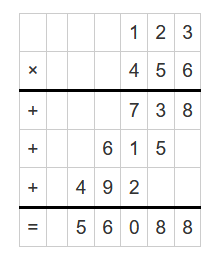Calculate the multiplication of two numbers:

#### Multiplication Calculator

Result:

 X * Y: 0

### What is Multiplication?

Multiplication is one of the basic arithmetic operations. It involves taking two (or more) numbers, and combining them to get a new number. This new number is called the product of the numbers which were multiplied. The symbol used to denote multiplication is “×” or “.”(dot).

For example, consider “4 × 5 = 20”. Here, × is the multiplication operator and 20 is the product.

There might be more than two numbers being multiplied at the same time. Consider “13 × 6 × 8 = 624”. Here, three numbers are being multiplied: 13, 6 and 8, × is the multiplication operator and 624 is the product.

### Properties of Multiplication

Given below are some important properties of multiplication.

Product – The number obtained after doing the multiplication of two or more numbers.

Multiplication operator – Denoted by “×” or “.”, this sign is an arithmetic operator which indicates the multiplication of two or more numbers.

Closure property – The product of two or more integers is always an integer. For example, 4 × 5 = 20. Here, 4 and 5 are integers, and so is their product, 20. The same is true for real numbers as well. For example, 4.1 × 3.5 = 14.35. Here, 4.1, 3.5 and their product 14.35 are all real numbers.

Commutative property – When the order of the numbers being multiplied is changed, the product does not change. For example, 4 × 5 = 20 and 5 × 4 = 20.

Associative property – When the groups of numbers being multiplied are changed, the product does not change. For example, (13 × 6) × 8 = 624 and 13 × (6 × 8) = 624.

Distributive property – If P, Q and R are three different numbers, then according to the distributive property of multiplication, P × (Q + R) = P × Q + P × R. For example, 3 × (4 + 5) = 3 × 4 + 3 × 5 = 12 + 15 = 27.

Identity property – When 1 is multiplied with any number, the product is that number itself. For example, 4 × 1 = 4.

Zero property – When 0 is multiplied with any number, the product is always zero. For example, 4 × 0= 0.

### Multiplication Rules

The numbers involved in the process of multiplication may not always be positive. Below are the rules followed for the multiplication of different kinds of numbers.

Positive number × positive number = positive number. For example, 4 × 5 = 2.

Negative number × negative number = positive number. For example, (-4) × (-5) = 20.

Positive number × negative number = negative number. For example, (- 5) × 4 = - 20, and (– 4) × 5 = -20.

### Steps for Multiplication

1) Long multiplication of integers:

When the numbers being multiplied are large or if there are more than two large number being multiplied, the multiplication process may become longer. For example, the multiplication of 123 and 456.

This can be done in the following steps.

• First, arrange on top of the other and align the digits according to place value. The number with more digits is usually written on top.
• Start the multiplication with the ones digit of the bottom number, and multiply it with the ones digit of the above number. Note down the answer.
• If the answer is greater than nine, the digit in ones place is written and the digit in tens place is carried to the number on the left.
• Continue from right to left. If a digit has been carried over, add it to the result of the multiplication and write down the answer.
• When the ones digit of the lower number has been multiplied with every digit of the upper number, move on to the tens digit of the lower number and repeat the process.
• Write the answers in a new row this time, but shift one place to the left.
• After all the digits of the lower number have been multiplied with the upper number, draw an answer line below the last row.
• Finally, use long addition to add up the numbers in each column from right to left.
• The example below demonstrates long multiplication of whole numbers.2) Long multiplication of decimal numbers:

If the multiplication involves decimal numbers, the process is slightly different.

• First, count the number of decimal numbers in the two numbers. Make a note of this for later.
• Ignore the decimal points for now, and align the number one on top of the other as if they are integers.
• Multiply these numbers using long multiplication described above.
• After this is complete, insert a decimal point in the product so it has the total number of decimal places of the numbers being multiplied (obtained in the first step).
• The example below demonstrates long multiplication of decimal numbers. Multiply 45.6 with 7.89There is a total of 3 decimal places in both the numbers.

Now, the product is written with 3 decimal places, as 359.784.

Therefore, 45.6 × 7.89 = 359.784

### Areas of application

Multiplication is basic arithmetic operation which we use quite frequently in daily life. Some examples are:

• When we need to find out the days in a week or weeks in a month, we multiply.
• While travelling, we need multiplication to find the approximate time to reach our destination when the distance is known.
• Multiplication is used in times tables, which is an essential building block for higher mathematics like fractions and algebra.
• For photography, we need multiplication to find angles, depth, perspective and distance, to name a few.

• Please check our interactive Multiplication table and learn further here